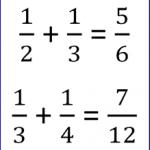Smartick is an online platform for children to master math in only 15 minutes a day

Jun16

## How to Solve Double Digit Division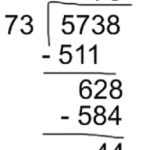In today’s post we are going to explain how to solve double digit division. Before beginning to learn how to solve double digit division, it is important that you become familiar with these terms, because we will use them later. Dividend: the number that is being divided. Divisor: the number by which the dividend is […]

Jun15

## Geometric Shape: Properties of a SquareToday, we’re going to take a look at a shape that you definitely know already, but maybe you aren’t familiar with all of its main characteristics. I’m talking about the square. Geometric Shape: Square The square is a geometric shape that belongs to the quadrilateral family because it has 4 sides. The 4 sides are […]

Jun13

## Kumon vs Smartick: Similarities and Differences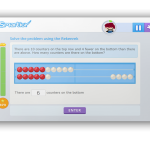Parents often ask what are the main differences between Smartick and other more traditional, paper-based methods (such as Kumon). The differences are substantial, both in the methodology, learning effectiveness and results. 1. Types of exercises Smartick does not come with preloaded exercises, nor worksheets with a fixed, preset sequence of exercises that are the same […]

Jun10

## Dividing a Decimal by a Whole Number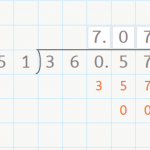Dividing a Decimal by a Whole Number Hello everyone! In this week’s post, we are going to talk about dividing a decimal by a whole number. You already know how to divide a whole number by a whole number by now, right? If not, check out our posts about dividing by a one-digit number here, […]

Jun09

## Properties of Multiplication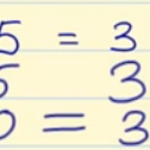The properties of multiplication are distributive, commutative, associative, removing a common factor and the neutral element. We are dedicating this post to examining the properties of multiplication, which are the following: Distributive property: The multiplication of a number by a sum is equal to the sum of the multiplications of this number by each one […]

Jun08

## Learn How to Subtract without Regrouping

Hello and welcome back! Today we are going to learn how to subtract without regrouping. Are you ready? Subtract Without Regrouping We’re going to follow a few steps. If you follow them well, you’ll learn how to subtract without regrouping in no time: You need to place the subtrahend below the minuend so that the ones-place […]

Jun06

## Smartick Chosen by MIT to Enhance Math Learning in the USIn 2015, MIT (Massachusetts Institute of Technology) and Smartick formed a partnership in order to accelerate our expansion into the North American market. The Global Internship Lab (G-Lab) selected our start-up to accelerate internationalization. Students and professors from one of the best MBA programs worldwide worked with us for four months to identify the challenges […]

Jun03

## Solve and Analyze Numerical Place Values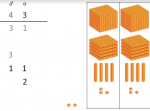Numerical Place Values What are the numerical place values? Why does a number’s value change according to its position? We’ll explain everything you need to know here about understanding mathematical algorithms by working with numerical place values. In this post, we are going to learn what numerical place value is, why it’s important and how […]